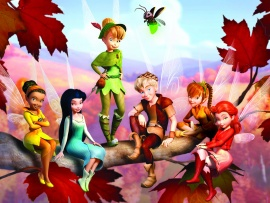WE USE COOKIES           ** FACEBOOK PAGE
Tinker Bell, dressed somewhat like Peter Pan, is posing with her other Fairie friends in this Disney wallpaper based upon the 2009 disney movie, Tinker Bell and the Lost Treasure.## Download Tinker Bell & Friends Wallpaper wallpaper | Leave a Comment

### function roundNumber(b,c){var a=Math.round(b*Math.pow(10,c))/Math.pow(10,c);return a}function resPrints(){var b=screen.width;var c=screen.height;var a=roundNumber(b/c,2);var d="Your display Resolution: <strong> "+b+" x "+c+" </strong> | Aspect Ratio:<strong> ";if(a==1.25||a==1.33){d+="Standard 4:3"}else{if(a==1.6||a==1.67){d+="Wide 8:5"}else{if(a==1.78){d+="HD 16:9"}else{if(a==1.76){d+="Mobile PSP"}else{if(a==0.75||(a==1.33&&b<=640)){d+="Mobile VGA"}else{if(a==0.6||(a==1.67&&b<=800)){d+="Mobile WVGA"}else{if(a==0.67||(a==1.5&&b==480)){d+="Mobile iPhone"}else{if(a==0.8){d+="Mobile Phone"}else{d="Unknown"}}}}}}}}d+="</strong>";document.write(d)}; resPrints();

Wallpaper details:
Added: Tuesday, 06 October 2009 20:06
Downloads: 96172
Sub Categories:
License: Free for personal wallpaper use only